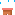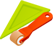Discovery Of Science# Speed

Click the picture, what are you see ?
All moving objects have speed. An object which moves faster travels a longer distance in a given time.

The speed of a moving object can be calculated if the distance travelled by the object and the time taken to travel the distance is known.

Units of speed: centimeters per second (cm/s), meters per second (m/s), kilometers per hour (km/h)

Examples:

a) A bus takes four hours to reach a destination which is 280km away.

Speed = 280 km / 4 h = 70km/h

b) A toy train takes 5 minutes to travel a distance of 30 cm.

Speed = 30cm / 5 min = 6 cm / min.